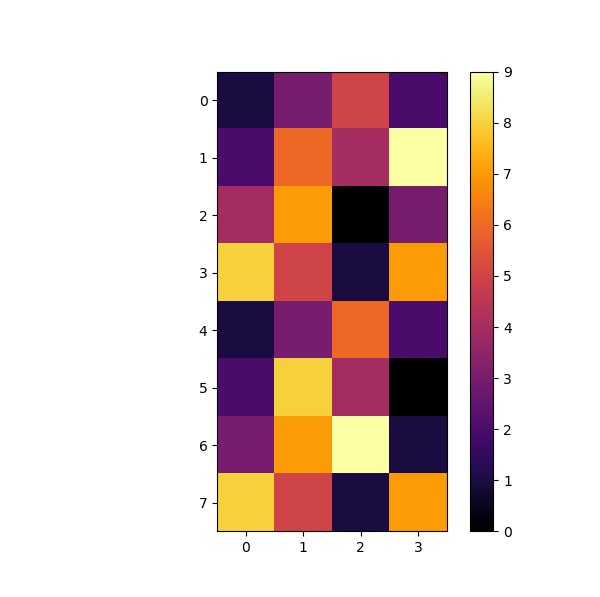``````import numpy as np
import matplotlib.pyplot as plt

#data为8x4的矩阵
data=np.array([[1,3,5,2],[2,6,4,9],[4,7,0,3],[8,5,1,7],[1,3,6,2],[2,8,4,0],[3,7,9,1],[8,5,1,7]])

plt.figure(figsize=(6, 6))
cmap=plt.cm.inferno#设置热力图的颜色样式
img=plt.imshow(data,cmap=cmap)
plt.colorbar()
``````2个回答Cody Pong 换成plt.figure(dpi=XX)也没有用，不管dpi是多少，出来的形状都是一样的。这个blog里更改dpi之后，只有横坐标的比例发生了变化......但我更改dpi后横纵坐标是一起变的......
3 个月之前 回复

plt.axis('tight')python使用scatter绘制散点图，如何一次绘制多组？

python 散点图绘制坐标轴异常

python plt 绘图 问题

![图片说明](https://img-ask.csdn.net/upload/201808/14/1534227583_383436.png) 如图， y 轴 刻度， 红框中间的那部分刻度是没用的，我也没设置中间这部分刻度，plt.yticks(y, [i for i in range(0, 401, 10)] + [i for i in range(2399, 2699, 100)])， 请问怎么能不显示这多余的刻度呢，感觉它自动增长的呢

python 用plt.title()怎么把图的标题放在图的下面？或者有其他的方法解决

python 用plt.title()怎么把图的标题放在图的下面？或者有其他的方法解决 ``` plt.figure(1,figsize=(12,8)) plt.bar(idx,team_season_3pt,label='场均出手' , color='deeppink') plt.bar(idx,team_season_3p,label='场均命中数', color='black') plt.scatter(idx,rate,label='命中率' , color='blue') plt.plot(idx,rate,linewidth=0.5,color='black',linestyle='-') plt.legend(bbox_to_anchor=(1,1)) plt.setp(plt.xticks(idx,tick),rotation=90) plt.title('三分球统计（常规赛）') plt.xlabel('年份-冠军球队') plt.show() ``` 现在想把图的标题放在图的正下方怎么修改呢

python用plotly绘制折线图为什么跑下来没有图

<div class="post-text" itemprop="text"> <p>如何更改用matplotlib绘制的图形大小?</p> </div> <p>转载于:https://stackoverflow.com/questions/332289/how-do-you-change-the-size-of-figures-drawn-with-matplotlib</p>

python 如何统计数据并绘制字母柱状图

python中用hist模块进行作图时，如何使不同色块之间有明显的线的区分，而不是一整块

1.问题描述如上 ``` import matplotlib.pyplot as plt import numpy.random as npr rn1 = npr.rand(500,3) plt.figure(figsize = (6,4)) plt.hist(rn1,bins=25,stacked=True) ``` 然后图片出来是这样的： ![图片说明](https://img-ask.csdn.net/upload/202002/22/1582357537_965159.png) 求助能不能让色块有明显的区别

fig = plt.figure(figsize=(2.5, 3.53557), dpi=180) ax1 = fig.add_subplot(711) norma = mpl.colors.Normalize(vmin=0, vmax=40) map = ax1.imshow(data112, interpolation='nearest', cmap=cmap, as pect='auto', norm=norma, origin='lower') font2 = {'family': 'Times New Roman', 'weight': 'normal', 'size': 30} plt.xlabel('Electron low energy range(1～22MeV)', font2, fontsize=4, labelpad=0.5) plt.ylabel('Pitch Angle(deg.)', font2, fontsize=3, labelpad=0.1) plt.xlim(0, 262) plt.ylim(0, 9) cb = fig.colorbar(mappable=map, cax=None, ax=ax1, pad=0.01, aspect=2, shrink=1.0, norm=norm, ticks=[0, 10, 20, 30]) cb.set_label('\$Counts(cm^-2s^-1Str^-1)\$', size=2) cb.ax.tick_params(labelsize=4, direction='in', right='True') ax7 = fig.add_subplot(717) ax7.plot(np.arange(0, len(Electron_counts)), Electron_counts, color='b', label='e', linewidth=0.4) ax7.plot(np.arange(0, len(Proton_counts)), Proton_counts, color='r', label='p', linewidth=0.4) plt.legend(bbox_to_anchor=(1.01, 0.5), loc=6, borderaxespad=0, fontsize=4) font2 = {'family': 'Times New Roman', 'weight': 'normal', 'size': 30} plt.xlabel('Electron low energy range(1～22MeV)', font2, fontsize=2, labelpad=0.5) plt.xlim(0, 262) plt.ylim(0, 340) plt.ylabel('\$Counts(cm^-2s^-1Str^-1)\$', font2, fontsize=2, labelpad=0.5) plt.yticks([0, 100, 200, 300], ['0', '100', '200', '300']) plt.show() ![图片说明](https://img-ask.csdn.net/upload/201908/17/1566030580_217227.png) ``` ```

python plt.show()总是无限弹出另存为窗口?

python在绘制图像时候，x轴的坐标未能显示

python在绘制图像时候，x轴的坐标未能显示。 显示的图片如下： ![图片说明](https://img-ask.csdn.net/upload/202004/15/1586948508_773451.png) 代码如下（本人用的是 jupyter notebook）： ``` import urllib.request import json import pygal import math from itertools import groupby # json_url = "https://raw.githubusercontent.com/muxuezi/btc/master/btc_close_2017.json" # req = urllib.request.urlopen(json_url) # json = req.read() # #将数据写入文件 # with open("E:/Jupyter/json收盘数据.json","wb") as f: # f.write(json) filename = "E:/Jupyter/json收盘数据.json" with open(filename) as f: btc_data = json.load(f) # #打印每一天的信息(获取数据过程) # for btc_dict in btc_data: # data = btc_dict["date"] # month = int(btc_dict["month"]) # week = int(btc_dict["week"]) # weekday = btc_dict["weekday"] # close = int(float(btc_dict["close"])) # print("{} is month {} week {},{},the close price is {}RMB".format(data,month,weekday,weekday,close)) #绘制收盘价折线图 dates = [] month = [] weeks = [] weekdays = [] close = [] #每一天信息 for btc_dict in btc_data: dates.append(btc_dict["date"]) month.append(int(btc_dict["month"])) weeks.append(int(btc_dict["week"])) weekdays.append(btc_dict["weekday"]) close.append(int(float(btc_dict["close"]))) #绘制收盘价对数变换价折线图 line_chart = pygal.Line(x_label_rotation = 20,show_minor_x_labels = True) line_chart.title = "收盘价" line_chart.x_label = dates N = 20 #x轴每隔20天显示一次 line_chart.x_labels_major = dates[::N] close_log = [math.log10(_) for _ in close] line_chart.add("收盘价",close_log) line_chart.render_to_file("收盘对数变换价折现图.svg") ```

python分组绘制箱线图的问题

python中的画图坐标问题

python3.7 读取csv文件某一行用plt画图坐标轴乱，不按数字大小排列怎么解决？

Python的多重饼图怎么绘制

python使用matplotlib.pyplot绘制折线图纵坐标（y轴）与实际图内坐标不符，请问是什么原因呢？

python 画图，用csv,怎么修改时间间隔和x轴间隔

fig.autofmt_xdate() # 设置x轴时间外观 ax1 = fig.add_subplot(1,1,1) autodate = mdate.AutoDateLocator() ax1.xaxis.set_major_locator(autodate) # 设置时间间隔 # 设置时间标签显示格式 dateFmt = mdate.DateFormatter('%y%m%d') ax1.xaxis.set_major_formatter(dateFmt) # 将x轴次刻度标签设置为61的倍数 xminorLocator = MultipleLocator(61) # 显示次刻度标签的位置,没有标签文本 ax1.xaxis.set_minor_locator(xminorLocator) #ax1.set_xticks() # 设置间隔 ax1.set_xlim(date2num('2018/01/02'),date2num('2018/01/29')) # 设置x轴范围 plt.xticks(rotation=90) # 显示日期旋转90度 plt.title(u'图') plt.plot(x,y, label='') plt.grid(True) plt.xlabel(u'日期') plt.ylabel(u'百分比') plt.legend(loc=2,prop={'family':'SimHei','size':12}) # loc=2 : upper left plt.savefig("图.png", dpi=100) plt.show()

python使用pandas处理绘图问题

4小时玩转微信小程序——基础入门与微信支付实战

4小时玩转微信小程序——基础入门与微信支付实战

Python可以这样学（第四季：数据分析与科学计算可视化）

Python可以这样学（第四季：数据分析与科学计算可视化）

javaWeb图书馆管理系统源码mysql版本

Java面试题大全（2020版）

Java8零基础入门视频教程

Java8零基础入门视频教程

Java基础知识面试题（2020最新版）

TTP229触摸代码以及触摸返回值处理

java jdk 8 帮助文档 中文 文档 chm 谷歌翻译

JDK1.8 API 中文谷歌翻译版 java帮助文档 JDK API java 帮助文档 谷歌翻译 JDK1.8 API 中文 谷歌翻译版 java帮助文档 Java最新帮助文档 本帮助文档是使用谷

Ubuntu18.04安装教程

Ubuntu18.04.1安装一、准备工作1.下载Ubuntu18.04.1 LTS2.制作U盘启动盘3.准备 Ubuntu18.04.1 的硬盘空间二、安装Ubuntu18.04.1三、安装后的一些工作1.安装输入法2.更换软件源四、双系统如何卸载Ubuntu18.04.1新的改变功能快捷键合理的创建标题，有助于目录的生成如何改变文本的样式插入链接与图片如何插入一段漂亮的代码片生成一个适合你的列...

HTML期末大作业

Python数据挖掘简易入门

Python数据挖掘简易入门

C++语言基础视频教程

C++语言基础视频教程

UnityLicence

UnityLicence

YOLOv3目标检测实战：训练自己的数据集

YOLOv3目标检测实战：训练自己的数据集

Python数据分析师-实战系列

YOLOv3目标检测实战系列课程

《YOLOv3目标检测实战系列课程》旨在帮助大家掌握YOLOv3目标检测的训练、原理、源码与网络模型改进方法。 本课程的YOLOv3使用原作darknet（c语言编写），在Ubuntu系统上做项目演示。 本系列课程包括三门课： (1)《YOLOv3目标检测实战：训练自己的数据集》 包括：安装darknet、给自己的数据集打标签、整理自己的数据集、修改配置文件、训练自己的数据集、测试训练出的网络模型、性能统计(mAP计算和画出PR曲线)和先验框聚类。 (2)《YOLOv3目标检测：原理与源码解析》讲解YOLOv1、YOLOv2、YOLOv3的原理、程序流程并解析各层的源码。 (3)《YOLOv3目标检测：网络模型改进方法》讲解YOLOv3的改进方法，包括改进1：不显示指定类别目标的方法 （增加功能） ；改进2：合并BN层到卷积层 （加快推理速度） ； 改进3：使用GIoU指标和损失函数 （提高检测精度） ；改进4：tiny YOLOv3 （简化网络模型）并介绍 AlexeyAB/darknet项目。

web网页制作期末大作业

Spring Boot -01- 快速入门篇（图文教程）

Spring Boot -01- 快速入门篇 今天开始不断整理 Spring Boot 2.0 版本学习笔记，大家可以在博客看到我的笔记，然后大家想看视频课程也可以到【慕课网】手机 app，去找【Spring Boot 2.0 深度实践】的课程，令人开心的是，课程完全免费！ 什么是 Spring Boot？ Spring Boot 是由 Pivotal 团队提供的全新框架。Spring Boot...